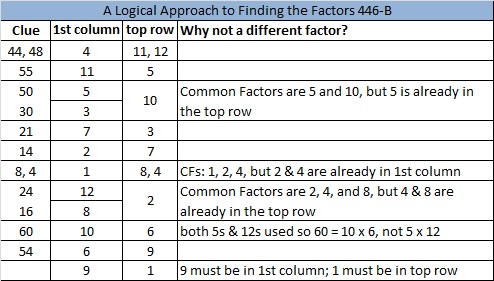# 301 and Level 5

• 301 is a composite number.
• Prime factorization: 301 = 7 x 43
• The exponents in the prime factorization are 1 and 1. Adding one to each and multiplying we get (1 + 1)(1 + 1) = 2 x 2 = 4. Therefore 301 has exactly 4 factors.
• Factors of 301: 1, 7, 43, 301
• Factor pairs: 301 = 1 x 301 or 7 x 43
• 301 has no square factors that allow its square root to be simplified. √301 ≈ 17.349Print the puzzles or type the factors on this excel file: 12 Factors 2014-11-17This site uses Akismet to reduce spam. Learn how your comment data is processed.